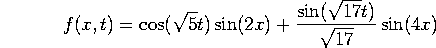M346 Final Exam Solutions
Given December 13, 2000

Problem 1.

Consider the vector space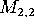of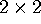matrices, let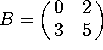. Consider the linear transformations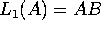and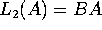.

a) Find the matrix of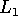relative to the basis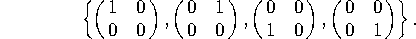We compute: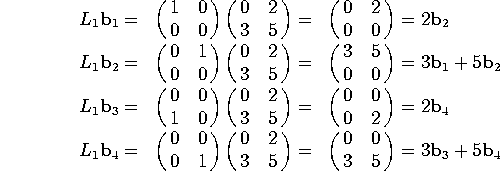so we have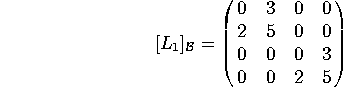b) Find the matrix of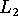relative to the same basis.

We compute: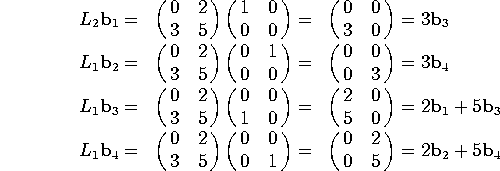so we have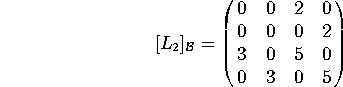Problem 2. Let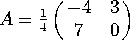.

a) Consider the equations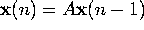, with A as above. What are the stable and unstable modes? What is the dominant eigenvector?

The eigenvalues are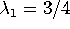and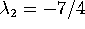, with eigenvectors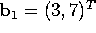and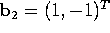. The first mode is stable since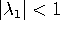, while the second is unstable since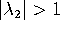. The dominant eigenvector is.

b) Consider the equations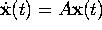, with A as above. What are the stable and unstable modes? What is the dominant eigenvector?

Now the question is whether the real part of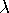is positive or negative. Since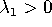, the first mode is unstable. Since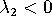, the second mode is stable. Now the dominant eigenvector is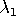.

Problem 3. Let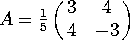. Which of the following are Hermitian? Which are unitary? Which are both? Which are neither?

Notice that the eigenvalues of A are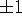, and the eigenvectors are orthogonal. You can see this by calculating them [they are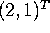and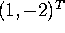], or from the fact that A is manifestly Hermitian. The various operations all give matrices with the same eigenvectors as A, but different eigenvalues. Since the eigenvectors are orthogonal, a matrix will be Hermitian if its eigenvalues are real, and unitary if its eigenvalues have norm one.

a) A

Both Hermitian and unitary, since 1 and -1 are both real and of norm 1.

b) A + I

Hermitian but not unitary, since 2 and 0 are real but not of norm 1.

c)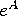Hermitian but not unitary, since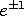are real but not of norm 1.

d)Unitary but not Hermitian, since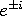are complex but of norm 1.

Problem 4. In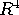with the standard inner product, consider the vectors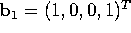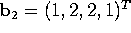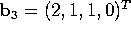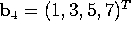. Apply Gram-Schmidt to turn this into an orthogonal basis for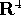.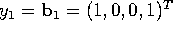.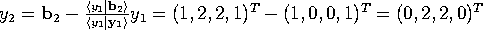.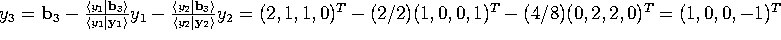.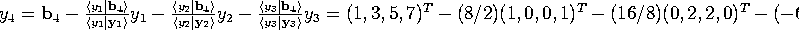.
(sorry...that got cut off by the latex2html program.  The final answer is y4=(0,-1,1,0)^T.

Problem 5. Consider a sequence of numbers satisfying the second order difference equation x(n) = 2 x(n-1) + 3 x(n-2) for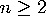.

a) Reduce this 2nd order difference equation to asystem of first order difference equations.

Let y(n)=x(n-1). Then x(n) = 2x(n-1) + 3y(n-1), and we have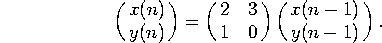b) Find the most general solution to the first order system.

The eigenvalues of the matrix are 3 and -1, with eigenvectors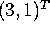and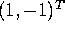, so the most general solution is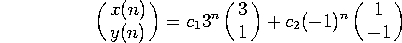c) From initial data x(0)=2, x(1)=2, find x(n) for all n.

We have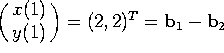, so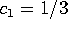and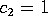. Thus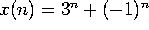.

Problem 6. Consider the nonlinear system of equations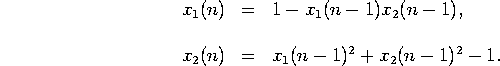a) Linearize this system of equations near the fixed point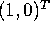.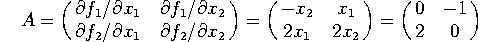Defining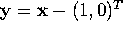, our linearized equations are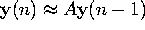.

b) Find the modes and determine which are stable and which are unstable.

The eigenvalues of A are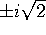, with eigenvectors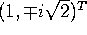. Since both eigenvalues are (in magnitude) bigger than 1, both modes are unstable.

c) Is the fixed pointstable?

And sois an unstable fixed point.

Problem 7. Diagonalize the matrix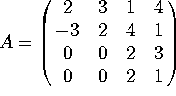Since it is block triangular, to find the eigenvalues you just need to diagonalize each block. The eigenvalues of the upper left block are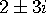, while the eigenvalues of the lower right block are 4 and -1. The eigenvectors are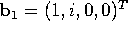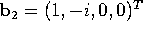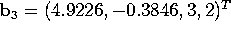,  b4=(1, 0, 1, -1)^T.  Computing the first two entries of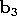is messy. I'll accept an answer of
(junk, junk, 3,2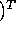.

Problem 8.

We wish to solve the differential equation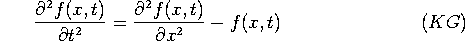on the interval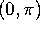with Dirichlet boundary conditions: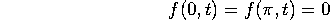for all t. [This is called the Klein-Gordon equation, and comes up in relativistic quantum mechanics. We have not studied this equation, but you can solve it using the same ideas that gave us standing waves solutions to the wave equation.]

a) Find the eigenvalues and eigenfunctions of the operator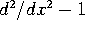(with Dirichlet boundary conditions).

We already know the eigenvalues and eigenvectors of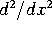, namely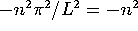and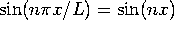. The eigenvalues ofare just one less (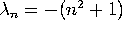) and the eigenvectors are the same.

b) Find the most general solution to (KG).

Let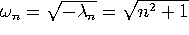. Then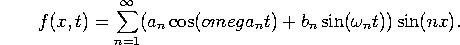c) Given the initial conditions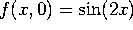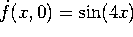, find f(x,t) for all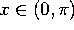and all t.

The only nonzero coefficients are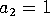and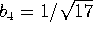, so